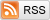# Don't Trust Asymptotics: Part I

March 25, 2013

Suppose I give you a sequence of real numbers $x_n$, and tell you that $\lim_n x_n = \infty$. What can you tell me about $x_{100}$? How about $x_{1,000,000}$?

The answer, of course, is "absolutely nothing". The limit of a sequence depends only on the tail behavior of that sequence, and not on any finite number of its terms. It might be that $x_{100}$ is a billion, and then $x_n$ continues on to infinity afterwards. Or maybe $x_{100}$ is negative a billion, and afterwards $x_n$ goes to infinity. Based on what I've told you about $x_n$, you have no idea. So if you really want to know how the sequence $x_n$ behaves, you need to make statements about its behavior for finite $n$.

Oftentimes, however, Statisticians seem to ignore this fact. A major theme within Statistics is asymptotics, which deals with the limiting distributions of random variables and the tail behavior of estimators. The main motivation for dealing in asymptotics, I think, is that it greatly simplifies the problem mathematically; unfortunately, it also makes the results technically vacuous, because they do not apply to any finite sample regardless of how large it is! In practice, what people will typically do is pretend that the asymptotic result applies to finite $n$, and hope that they aren't too far off.

But asymptotic properties do not always agree with finite sample properties: As my example for Part I of this series, consider the following simple problem: We have $X_1, \ldots, X_n$ which are iid normally distributed with mean $\mu$ and known variance 1, and we wish to estimate the mean $\mu$. The loss function we use is the usual squared error loss, so that the risk of the estimator $\hat{\mu}$ of $\mu$ is simply $$R(\mu, \hat{\mu}) = E_{\mu}\left[\left(\hat{\mu} - \mu\right)^2\right].$$

Of course, the usual thing to do would be to just use the sample mean $\bar{X}_n = \frac{1}{n}\sum_{i=1}^n X_i$ as an estimate of $\mu$. And, indeed, the sample mean has pretty much every good finite-sample property you could ask for: It is the best unbiased estimator, the minimum risk equivariant estimator, and it's also minimax and admissible. Since the sample mean is unbiased, its risk is just its variance, namely $1/n$ for every $\mu$, which achieves the Cramer-Rao lower bound.

On one of my homeworks last quarter, though, I was asked to consider the following interesting estimator of the mean: $$\hat{\mu}\left(X_1, \ldots, X_n\right) = \begin{cases} 0, &-n^{-0.25} \le \bar{X}_n \le n^{-0.25} \\ \bar{X}_n, &\mbox{otherwise} \end{cases}$$

$\hat{\mu}$ is just the sample mean, except that if the sample mean is too close to zero, $\hat{\mu}$ gets shrunk to zero. It's pretty easy to prove that $$\sqrt{n}\left(\hat{\mu} - \mu\right) \overset{D}{\to} \mathcal{N}\left(0, v(\mu)\right)$$

where $v(\mu) = 1$ if $\mu \ne 0$ and $v(0) = 0$. (The basic idea behind the proof is that as $n$ grows larger, the probability that $\bar{X}_n$ falls into the shrink-to-zero region goes to 1 or 0, depending on whether $\mu = 0$ or not). By comparison, the analogous statement for the sample mean has $v(\mu) = 1$ for all $\mu$. So both estimators are asymptotically unbiased, but $\hat{\mu}$ has lower asymptotic variance! Asymptotically, at least, $\hat{\mu}$ is the better estimator.

(If you're not impressed about $\hat{\mu}$ only being better at one point, note that the same shrinking trick would definitely still work in finitely many places, so you could have an asymptotically unbiased estimator with asymptotic variance zero under all means that are representable as 64-bit doubles, and one everywhere else. Since we always use floats or doubles for our calculations anyway, this is a great estimator, right?)

To compare the sample mean and shrunk sample mean for finite sample data, I actually calculated and plotted the risk function of the shrinkage estimator $\hat{\mu}$ for $n \in \{1, 16, 81, 256, 625, 1296\}$. (I posted the code here.) In the following plots, the flat, green line is the risk function for the ordinary sample mean, while the humped, blue line is the risk function for the sample mean with shrinking, (although note the different y-axis scales). You can see how the risk function for shrinkage estimator is about the same as the risk of the sample mean when $\mu$ is far from zero, (since shrinking is rare), is lowest when $\mu$ is very near zero, (since the sample mean gets shrunk to near the true $\mu$), and spikes up quite high when $\mu$ is only somewhat near zero, (since the sample mean is mistakenly shrunk to zero fairly often).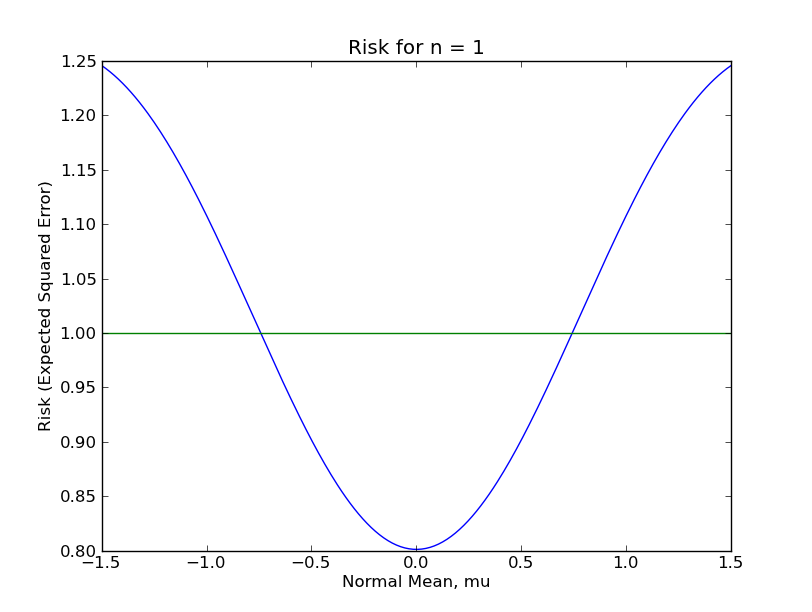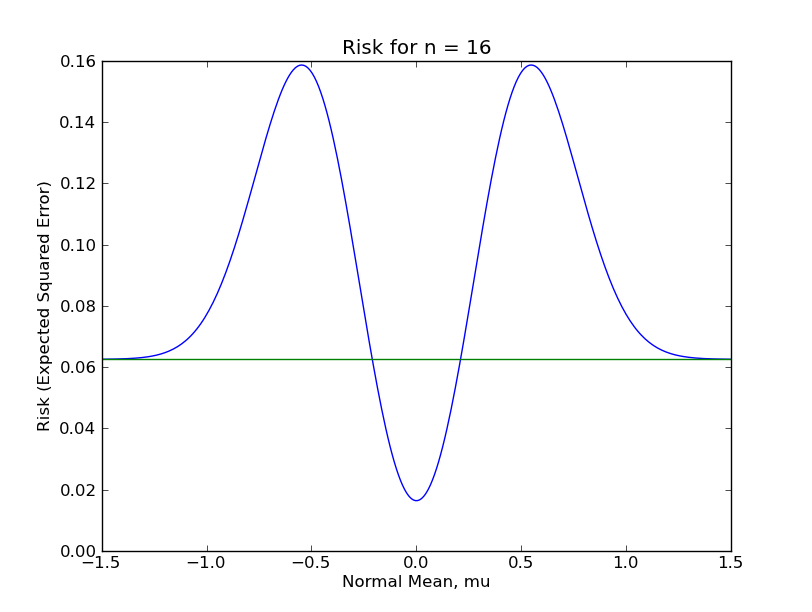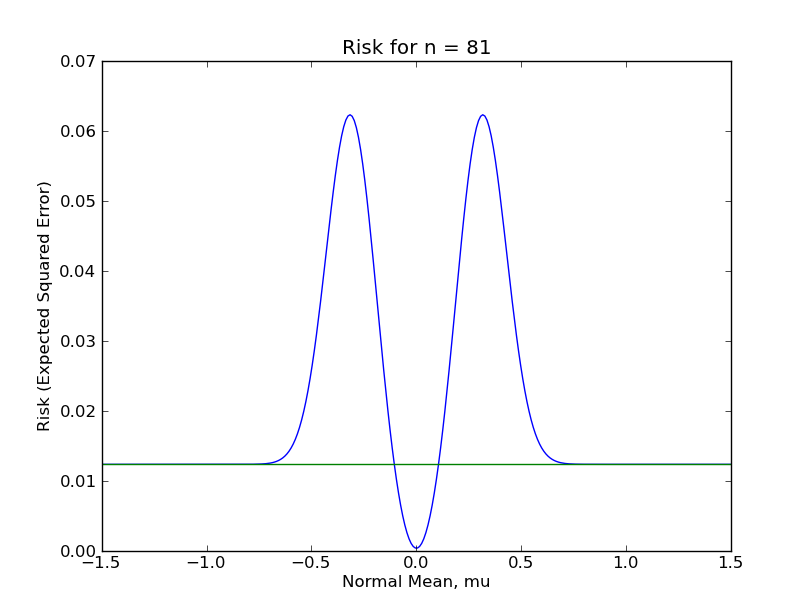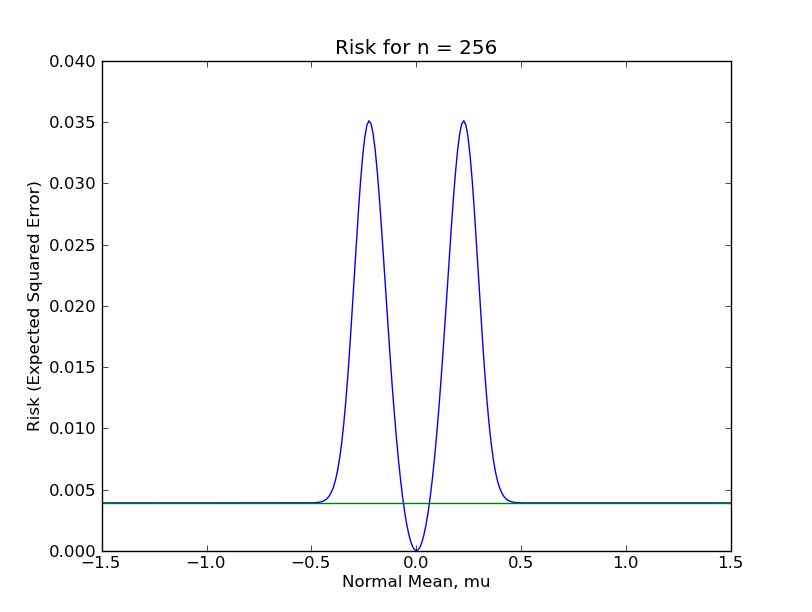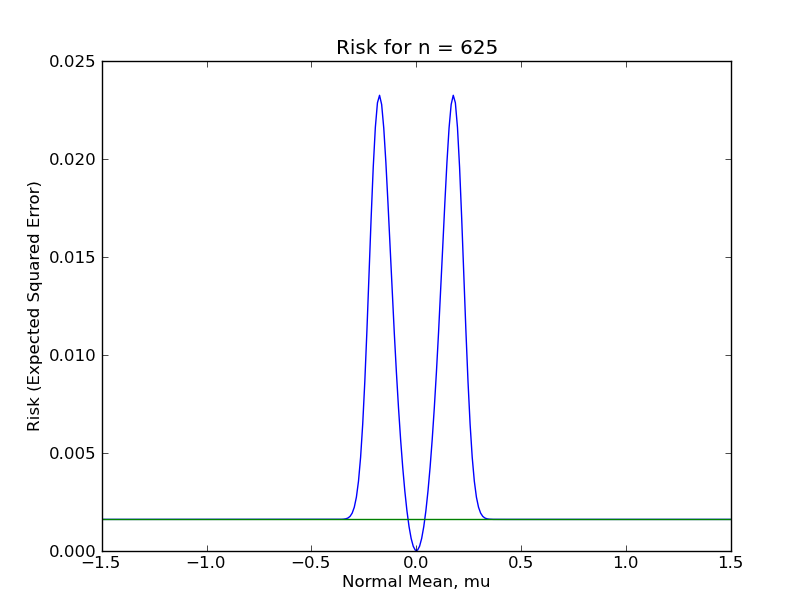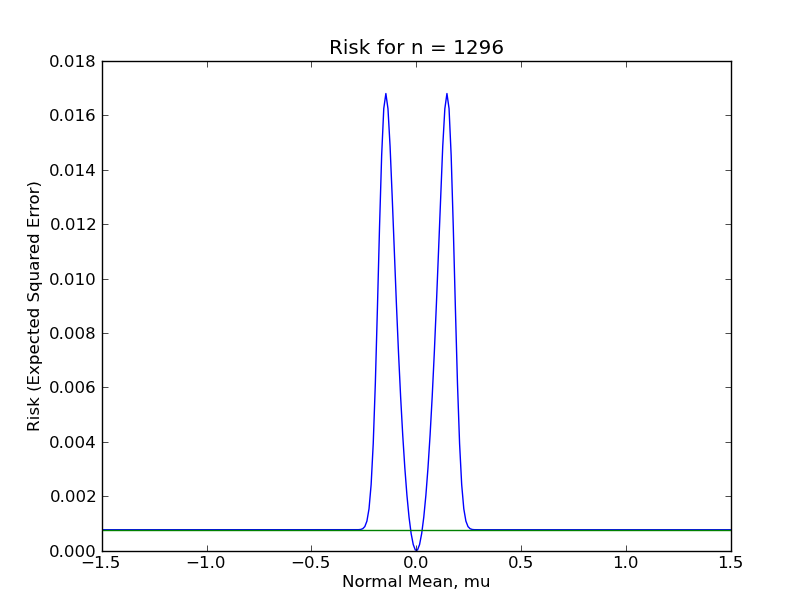From these plots, it should be clear that the shrinkage estimator is a far inferior estimator to the sample mean under almost any reasonable circumstance. This is because there are large regions of the parameter space in which the shrinkage estimator performs terribly. Even for $n = 1296$, which most Statisticians would consider a "large sample", the sup-risk is about $0.017$, which for comparison is the risk of the sample mean with only 59 observations.

The discrepancy between this estimator's poor finite-sample behavior and great asymptotic behavior should, I think, help convince us to be wary of asymptotic results. Asymptotic results do not say anything about finite samples, which is of course the only sort of sample that ever occurs in real life. We should therefore consider asymptotic results as mathematical curiosities that are sometimes close to reality, but other times arbitrarily far from it.